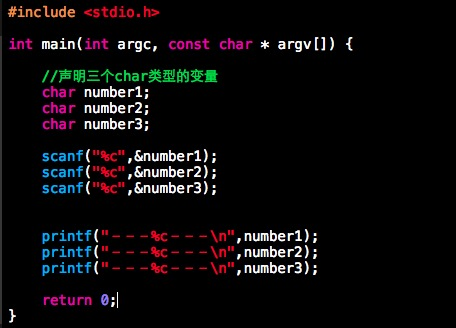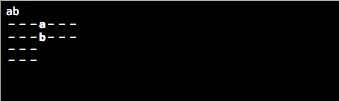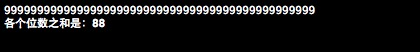# C语言Scanf函数剖析以及数字求和算法

int number;
scanf(“%d”,&number);／／请用户输入一个数字

1.控制台一次输入：12334（）
2.敲下回车，告诉计算机，输入结束（若不按回车需要用户不断输入）int number , temp , sum = 0;
scanf(“%d”,&number);
while(number!=0)
{
temp = number %10;
number /= 10;
sum += temp;
}
printf(“各个位数的和是%d”,sum);

char temp;
int sum = 0;
while(1){
temp = getchar(); －－－－－//从缓存中读取一个字符出来
if(temp ==’\n’) －－－－－ //循环结束的判断条件
break;
else
sum +=(temp-48);－－－－－//将字符转化为数字，进行求和
}
printf(“各个位数之和是%d\n”,sum);